#### Class B Amplifiers, Physics tutorial

Introduction:

Class B Amplifier utilizes two complimentary transistors (NPN and PNP) for each half of the output waveform. One transistor for positive half of waveform and another for negative half of waveform. This means that each transistor spends half of its time in Active region and half its time in Cut-off region. Class B operation has no DC bias voltage instead transistor only conducts when input signal is greater than base-emitter voltage and for silicon devices is approx 0.7v. Thus, at zero input there is zero output. This then results in only half the input signal being presented at amplifiers output giving greater efficiency as shown below.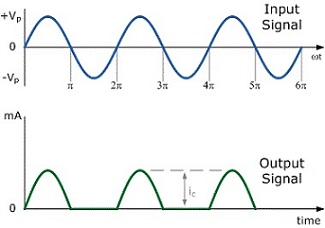As output transistors for each half of waveform, both positive and negative, needs a base-emitter voltage greater than 0.7v required for bipolar transistor to begin conducting, lower part of output waveform that is below this 0.7v window will not be reproduced correctly resulting in the distorted area of output waveform as one transistor turns OFF waiting for other to turn back ON. This kind of distortion is known as Crossover Distortion.

Class B amplifier operation:

Class B operation is provided when dc bias leaves transistor biased just off, transistor turning on when ac signal is applied. This is basically no bias, and transistor conducts current for only one-half of cycle. To get output full cycle of signal, it is essential to utilize two transistors and have each conduct on opposite half- cycle, combined operation giving full cycle of output signal.

As one part of circuit pushes signal high during one half-cycle and other part pulls signal low during other cycle, circuit is referred to as the push-pull circuit. The ac input signal is applied to push-pull circuit, with each half operating on alternate half-cycle, load then receiving a signal for full ac cycle. Power transistor utilized in push-pull circuit are able to deliver desired power to load, and class B operation of the transistors gives greater efficiency than was possible using single transistor in class A operation.

Input (DC) Power:

The power supplied to load by the amplifier is drawn from power supply (or power supplies which gives input or dc power. Amount of this input power can be computed using

Pi(dc) = VCCIdc

Where Idc is average or dc current drawn from power supplies. In class B operation, current drawn from single power supply has form of the full-wave rectified signal, while that drawn from two power supplies has form of the half-wave rectified signal from each supply.

In either case, value of average current drawn can be stated Idc = (2/π)l(p)

Where I(p) is peak value of output current waveform. Using Eq. in power input equation results in

Pi(dc) = VCC((2/π)l(p))

Output (ac) power:

The power delivered to load (generally referred to as resistance, RL) can be computed using any one of number of equations. If one is using rms meter to compute voltage across load, output power can be computed as

P0(ac) = V2L(rms)/RL

P0(ac) = V2L(p-p)/8RL = V2L(p)/2RL

If one is using oscilloscope, peak-to-peak, output voltage computed can be utilized. The larger the rms or peak output voltage, the larger the power delivered to load.

Efficiency:

Efficiency of class B amplifier can be computed using basic equation: %η = P0(ac)/Pi(dc) x 100%

Using Equations in efficiency equation above results in

%η = P0(ac)/Pi(dc) x 100% = [V2L(p)/2RL/(VCC((2/π)I(p)))] X 100% = πVL(p)/4VCC

(using I(p) = VL(p)/RL). Equation shows that larger the peak voltage, the higher the circuit efficiency, up to maximum value when VL(p) = VCC, this maximum efficiency then being

Maximum efficiency = π/4 x 100% = 78.5%

Power Dissipated by Output Transistors:

The power dissipated (as heat) by output power transistors is difference between input power delivered by supplies and output power delivered to load.

P2Q = Pi(dc) - P0(ac)

Where P2Q is power dissipated by two output power transistors. Dissipated power handled by each transistor is then

PQ = P2Q/2

Class B Amplifier Circuit:

The numbers of circuit arrangements for obtaining class B operation are possible. Input signals to amplifier could be single signal, circuit then providing two different output stages, each operating for one-half the cycle. If input is in form of two opposite polarity signals, two similar stages could be utilized, each operating on alternate cycle due to input signal. One way of obtaining polarity or phase inversion is using transformer, transformer-coupled amplifier having being popular for very long time. Opposite polarity inputs can simply be obtained using op-amp having two opposite outputs or using few op-amp stages to get two opposite polarity signals. The opposite polarity operation can also be attained using single input and complementary transistors (npn and pnp or nMOS and pMOS)

Phase-splitter Circuits diagram shows different ways to get phase-inverted signals from the single input signals. The center-tapped transformer to give opposite phase signals. If transformer is accurately center- tapped, two signals are exactly opposite in phase and are of same magnitude. Circuit uses the BJT stage with in-phase output from emitter and opposite phase output from collector. If gain is made almost 1 for each output same magnitude results. Probably most common would be using op-amp stages, one to give the inverting gain of unity and other non-inverting gain of unity, to give two outputs of same magnitude but of opposite phase.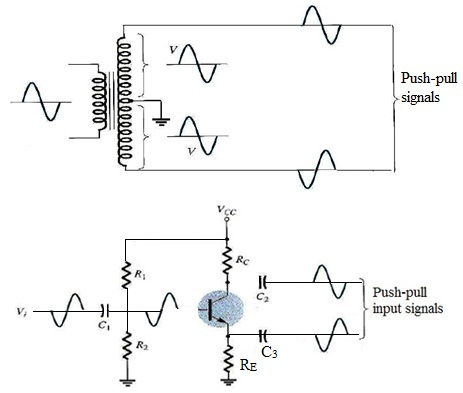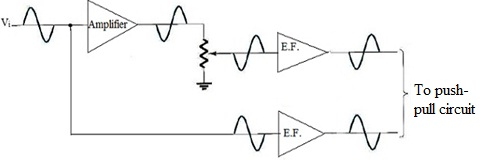Class AB Amplifiers:

Class AB Amplifier is the compromise between Class A and Class B configurations above. While Class AB operation still utilizes two complementary transistors in its output stage a very small biasing voltage is applied to Base of transistor to bias it close to Cut- off region when no input signal is present. The input signal will cause transistor to operate as normal in its Active region thereby reducing any crossover distortion. The small Collector current will flow when there is no input signal but it is much less than that for Class A amplifier configuration. This means then that transistor will be ON for more than half a cycle of waveform. This kind of amplifier configuration enhances both efficiency and linearity of amplifier circuit compared to Class A.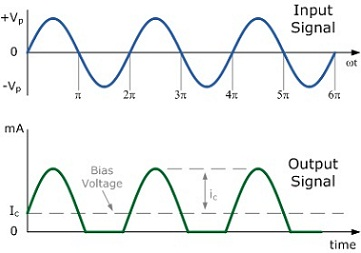The class of operation for the amplifier is very significant as it finds both efficiency and amount of power that amplifier consumes and dissipates in form of wasted heat, that may also need larger power transistors, more costly heat sinks, cooling fans, or even an increase in size of power supply needed to deliver extra power needed by amplifier. Power converted in heat from transistors, resistors or any other component makes any electronic circuit unproductive and will result in premature failure of device. Basically, Class A amplifier gives more linear output meaning that it has, Linearity over a larger frequency response.

Transformer Less Class AB Push-Pull Amplifier:

The base-emitter voltage to be greater than 0.7v for the silicon bipolar transistor to begin conducting, so if we were to replace two voltage divider biasing resistors connected to base terminals of transistors with two silicon Diodes, biasing voltage applied to transistors would now be equal to forward voltage drop of diode. Two diodes are usually known as Biasing Diodes or Compensating Diodes and are chosen to match characteristics of matching transistors.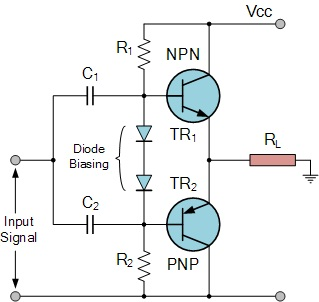Class AB Amplifier circuit is the compromise between Class A and Class B configurations. This very small diode biasing voltage causes both transistors to somewhat conduct even when no input signal is present. The input signal waveform will cause transistors to operate as normal in their active region thereby eliminating any crossover distortion. The small collector current will flow when there is no input signal but it is much less than that for Class A amplifier configuration. This signifies then that transistor will be ON for more than half a cycle of waveform but much less than full cycle. Amount of diode biasing voltage present at base terminal of transistor can also be increased in multiples by adding additional diodes in series.

Crossover Distortion:

One of the main drawbacks of the Class A Amplifier is its low full power efficiency rating. But we can enhance amplifier and almost double its efficiency simply by changing output stage of amplifier to the Class B push-pull type configuration. Though, this is great from efficiency viewpoint, but most modern Class B amplifiers are transformer less or complementary types with two transistors in their output stage. This results in one major fundamental problem with push-pull amplifiers in that two transistors don't combine together fully at output both halves of waveform because of their unique zero cut-off biasing arrangement. This problem takes place when signal changes or crosses-over from one transistor to other at zero voltage point it produces amount of distortion to output wave shape. This result in the condition which is commonly known as Crossover Distortion.

Crossover Distortion produces the zero voltage flat spot or dead band on output wave shape as it crosses over from one half of waveform to other. Reason for this is that transition period when transistors are switching over from one to other, doesn't stop or begin exactly at zero crossover point therefore causing small delay between first transistor turning OFF and second transistor turning ON. This delay results in both transistors being switched OFF at same instant in time producing an output wave shape as shown below.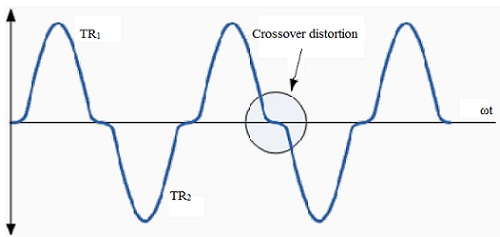In order that there must be no distortion of output waveform we should suppose that each transistor begins conducting when its base to emitter voltage rises just above zero, but we know that this is not true since for silicon bipolar transistors base voltage should reach at least before transistor begins to conduct thereby producing this flat spot. This crossover distortion effect also decreases overall peak to peak value of output waveform causing maximum power output to be reduced.

This effect is less pronounced for large input signals as input voltage is generally fairly large but for smaller input signals it can be more harsh causing audio distortion to amplifier.

Pre-biasing Output: Problem of Crossover Distortion can be reduced significantly by applying small forward base bias voltage (same idea as seen in Transistor tutorial) to bases of two transistors via center-tap of input transformer; therefore transistors are no longer biased at zero cut-off point but instead are Pre-biased at level found by this new biasing voltage.

This kind of resistor pre-biasing causes one transistor to turn ON exactly at same time as other transistor turns OFF as both transistors are now biased slightly above original cut-off point. Though, to achieve this bias voltage should be at least twice that of normal base to emitter voltage to turn ON transistors. This pre- biasing can also be employed in transformer less amplifiers which use complementary transistors by just replacing two potential divider resistors with Biasing Diodes.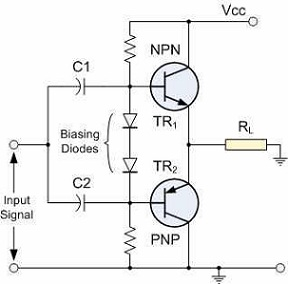This pre-biasing voltage either for transformer or transformer less amplifier circuit has effect of moving amplifiers Q-point past original cut-off point therefore allowing each transistor to work within its active region for slightly more than half or 180o of each half cycle. In other words 180o+ Bias. This then generates amplifier circuit usually known as a Class AB Amplifier.

Class C and class D amplifiers:

Though class A, class B and class AB amplifiers are most utilized as power amplifiers, class D amplifiers are popular due to their very high efficiency.

Class C Amplifier:

A class C amplifier is biased to operate for less than 1800 of input signal cycle. Tuned circuit in output, though, will give full cycle of output signal for fundamental or resonant frequency of tuned circuit (L and C tank circuit) of output. This kind of operation is thus limited to use at one fixed frequency, as takes place in communications circuit, for instance. Operation of the class C circuit is not intended mainly for large-signal or power amplifiers.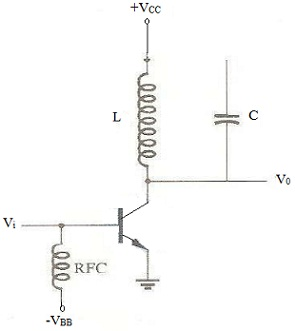Class D Amplifier:

Class D amplifier is developed to operate with digital or pulse-type signals. The efficiency of over 90% is attained using this kind of circuit, making it quite attractive in power amplifiers. It is essential, though, to convert any input signal to pulse-type waveform before using it to drive the large power load and convert signal back to the sinusoidal-type signal to recover original signal. D signal and then convert back to sinusoidal-type signal using low- pass filter. As amplifier's transistor devices utilized to give output are essentially either off or on, they give current only when they are turned on, with little power loss because of their low on-voltage. As most of the power applied to amplifier is transferred to load, efficiency of circuit is classically very high. Power MOSFET devices have been quite popular as driver devices for class D amplifier.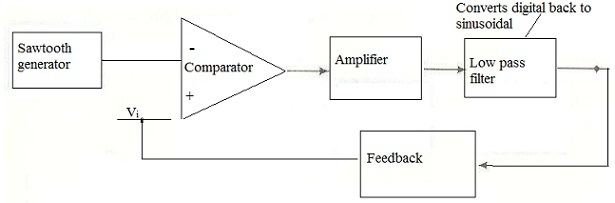Tutorsglobe: A way to secure high grade in your curriculum (Online Tutoring)

Expand your confidence, grow study skills and improve your grades.

Since 2009, Tutorsglobe has proactively helped millions of students to get better grades in school, college or university and score well in competitive tests with live, one-on-one online tutoring.

Using an advanced developed tutoring system providing little or no wait time, the students are connected on-demand with a tutor at www.tutorsglobe.com. Students work one-on-one, in real-time with a tutor, communicating and studying using a virtual whiteboard technology.  Scientific and mathematical notation, symbols, geometric figures, graphing and freehand drawing can be rendered quickly and easily in the advanced whiteboard.

Free to know our price and packages for online physics tutoring. Chat with us or submit request at info@tutorsglobe.com